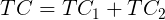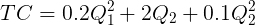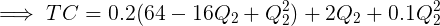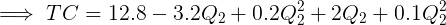# When a factory is operating in the short run.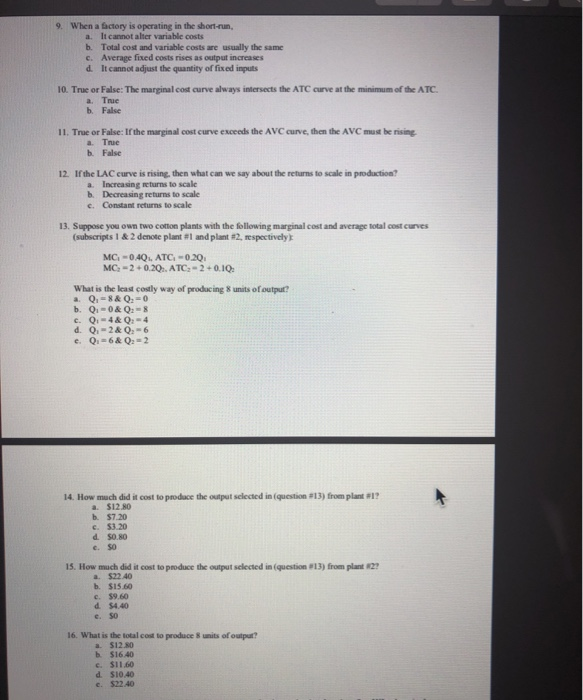9. When a factory is operating in the short-run. a. It cannot alter variable costs b Total cost and variable costs are usually the same c. Average fixed costs rises as output increases d. It cannot adjust the quantity of fixed inputs 10. True or False: The marginal cost curve always intersects the ATC curve at the minimum of the ATC a Tree b. False 11. True or False: If the marginal cost curve exceeds the AVC curve, then the AVC must be rising a True False 12. If the LAC curve is rising, then what can we say about the returns to scale in production? a. Increasing returns to scale b. Decreasing returns to scale c. Constant returns to scale 13. Suppose you own two cotton plants with the following marginal cost and average total cost curves (subscripts 1 & 2 denote plant #1 and plant #2, respectively MC -0.4Q.. ATC -0.20 MC-2 +0.20.. ATC -2 +0.10. What is the least costly way of producing 8 units of output? a 0, & 0. =0 b. Q.-0&Q28 c. 0. 4 & 4 d. 0.-2& 0, 6 e. Q. 6 & O 2 14. How much did it cost to produce the output selected in question 813) from plant #l? a S1280 b. \$7.20 \$3.20 d S0.80 e. SO 15. How much did it cost to produce the output selected in question 13) from plant 2? a \$22.40 b. \$15.60 c. \$9.60 16. What is the total cost to produce units of output? S12.80 b \$16.40 \$11.60 d \$10.40 c. \$22.40

9. When a firm is operating in the short run
Option D.it cannot change its quantity of fixed input.
In the short run the firms cannot change the fixed input. While
in the long run it can change all the inputs.
10. The MC curve always intersects
the ATC at the minimum of the ATC.
True.
11. If the marginal cost exceeds the
Minimum average variable cost then AVC must be rising.
True.
12. If LAC is rising then it
exhibits
Decreasing returns to scale.
13 to 16.

Note: Q1 + Q2 = 8
=> Q1 = 8 – Q2
Plug in this in the TC function

We have to minimize the TC function.
Differentiating TC wrt Q2 we get
MC = – 1.2 + 0.6Q2
Now equate it to zero
– 1.2 + 0.6Q2 = 0
=> 0.6Q2 = 1.2
=> Q2 = 2 units
Hence, Q1 = 8 – Q2 = 8 – 2 = 6
units
13. Q1 = 6; Q2 = 2
14. \$ 7.20
15. \$ 4.40
16. \$ 11.60
Q1 = 6 and Q2 =2
Plant 1 TC = Q1 × ATC1 = Q1 ×
0.2Q1
= 6 × 0.2×6 = \$ 7.20
Plant 2, TC2 = (2 + 0.1Q2)Q2 = (2 +
0.1×2) ×2 = (2+0.2)×2
= \$ 4.40
TC = 7.20 + 4.40 = \$ 11.60